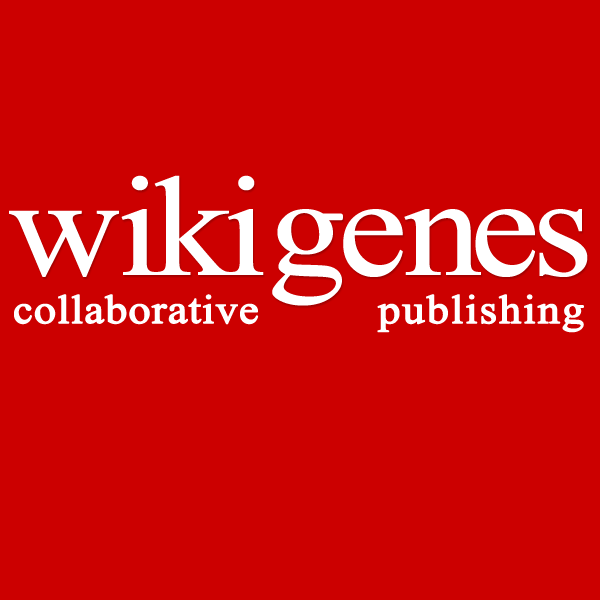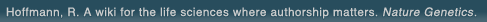# The world's first wiki where authorship really matters (Nature Genetics, 2008). Due credit and reputation for authors. Imagine a global collaborative knowledge base for original thoughts. Search thousands of articles and collaborate with scientists around the globe.

wikigene or wiki gene protein drug chemical gene disease author authorship tracking collaborative publishing evolutionary knowledge reputation system wiki2.0 global collaboration genes proteins drugs chemicals diseases compound
Hoffmann, R. A wiki for the life sciences where authorship matters. Nature Genetics (2008)# Effects of molecular structure on the stability of a thermotropic liquid crystal. Gas electron diffraction study of the molecular structure of phenyl benzoate.

As a model of the core of molecules forming liquid crystals, the molecular structure of phenyl benzoate (Ph-C(=O)-O-Ph) at 409 K was determined by gas electron diffraction, and the relationship between the gas-phase structures of model compounds and the nematic-to-liquid transition temperatures was studied. Structural constraints were obtained from RHF/6-31G ab initio calculations. Vibrational mean amplitudes and shrinkage corrections were calculated from the harmonic force constants given by normal coordinate analysis. Thermal vibrations were treated as small-amplitude motions, except for the phenyl torsion, which was treated as a large-amplitude motion. The potential function for torsion was assumed to be V(phi(1),phi(2)) = V(12)(1 - cos 2phi(1))/2 + V(14)(1 - cos 4phi(1))/2 + V(22)(1 - cos 2phi(2))/2, where phi(1) and phi(2) denote the torsional angles around the C-Ph and O-Ph bonds, respectively. The potential constants (V(ij)()/kcal mol(-)(1)) and the principal structure parameters (r(g)/A, angle(alpha)/deg) with the estimated limits of error (3sigma) are as follows: V(12) = -1.3 (assumed); V(14) = -0.5(9); V(22) = 3.5(15); r(C=O) = 1.208(4); r(C(=O)-O) = 1.362(6); r(C(=O)-O) - r(O-C) = -0.044 (assumed); r(C(=O)-C) = 1.478(10); <r(C-C)> = 1.396(1); angleOCO = 124.2(13); angleO=CC = 127.3(12); angleCOC = 121.4(22); ( angleOCC(cis) - angleOCC(trans))/2 = 3.0(15); ( angleC(=O)CC(cis) - angleC(=O)CC(trans))/2 = 4.8(17), where < > means an average value and C-C(cis) and C-C(trans) bonds are cis and trans to the C(=O)-O bond, respectively. The torsional angle around the O-Ph bond was determined to be 64(+26,-12) degrees. An apparent correlation was found between the contributions of the cores to the clearing point of liquid crystals and the gas-phase structures of model compounds of the cores of mesogens, i.e., phenyl benzoate, trans-azobenzene (t-AB), N-benzylideneaniline, N-benzylideneaniline N-oxide (NBANO), trans-azoxybenzene (t-AXB), and trans-stilbene. The structures of t-AB, NBANO, and t-AXB have been obtained by our research group.